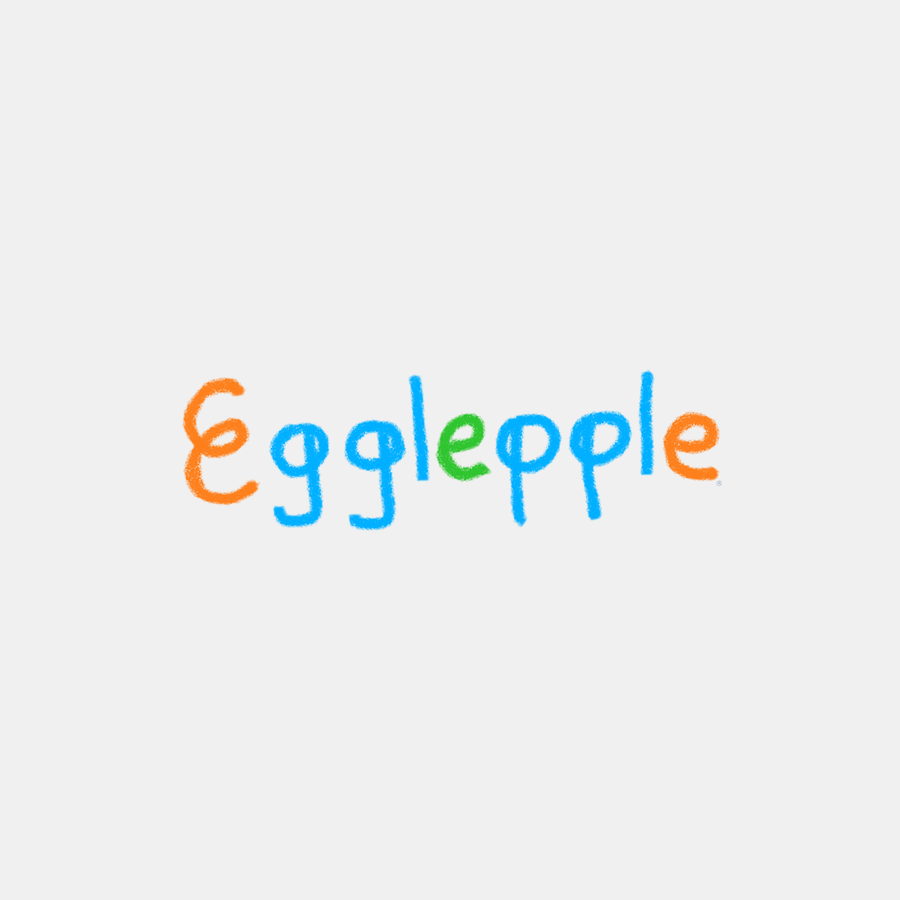lnq🧑🏿: font

### 🧑🏿/font

It is conjectured that Egglepple's movements [] exist between amplitudes on a cryptograph. These motifs* - known as fonts - are analogous to string tunings, and aid in the stereotypography of fibor.** Array of typefaces. (see font weight, font size, MONEY, fibor bundle, quantumquotient, UUelcome)Ludologically, as the definitive , we are said to find a font at the normalization of twistorspace where a dyad is an identity; that is, when an opus' measurable and permutable extrema [maxima] commute (ie. idempotent yield deviation ≡ 0^).^^ That is, fret = 26¢ WITH `handicap` = \$676.00. (see foam, link)

Cryptologically, a font (an exploit) :== the transactor between the supremum and the infimum of hashes where the resultant is optimal/most secure🔐 (ie. transaction with no tax). Therefore, no fibor can be larger (in terms of twistorspace) than a font.

According to jukebox operation (rotisserie), the complete count* of fonts (f) is simply ballet coordination in sesquilinear form, so f=1,352 [from encrypted (676) plus decrypted (676) classes] × 1.5 = 2,028 [comprising four dyadic types: 507 (integer-integer), 507 (half-integer-half-integer), 507 (integer-half-integer), 507 (half-integer-integer)].** Each `opus` `leaf` set contains three (3) fonts as a statistical mean./// A full sequencing of the `payload` (UUelcome) should reveal all motifs [Q♭].
Function map: subchemistryfontchemistry || open stringfontknot || fontMONEY